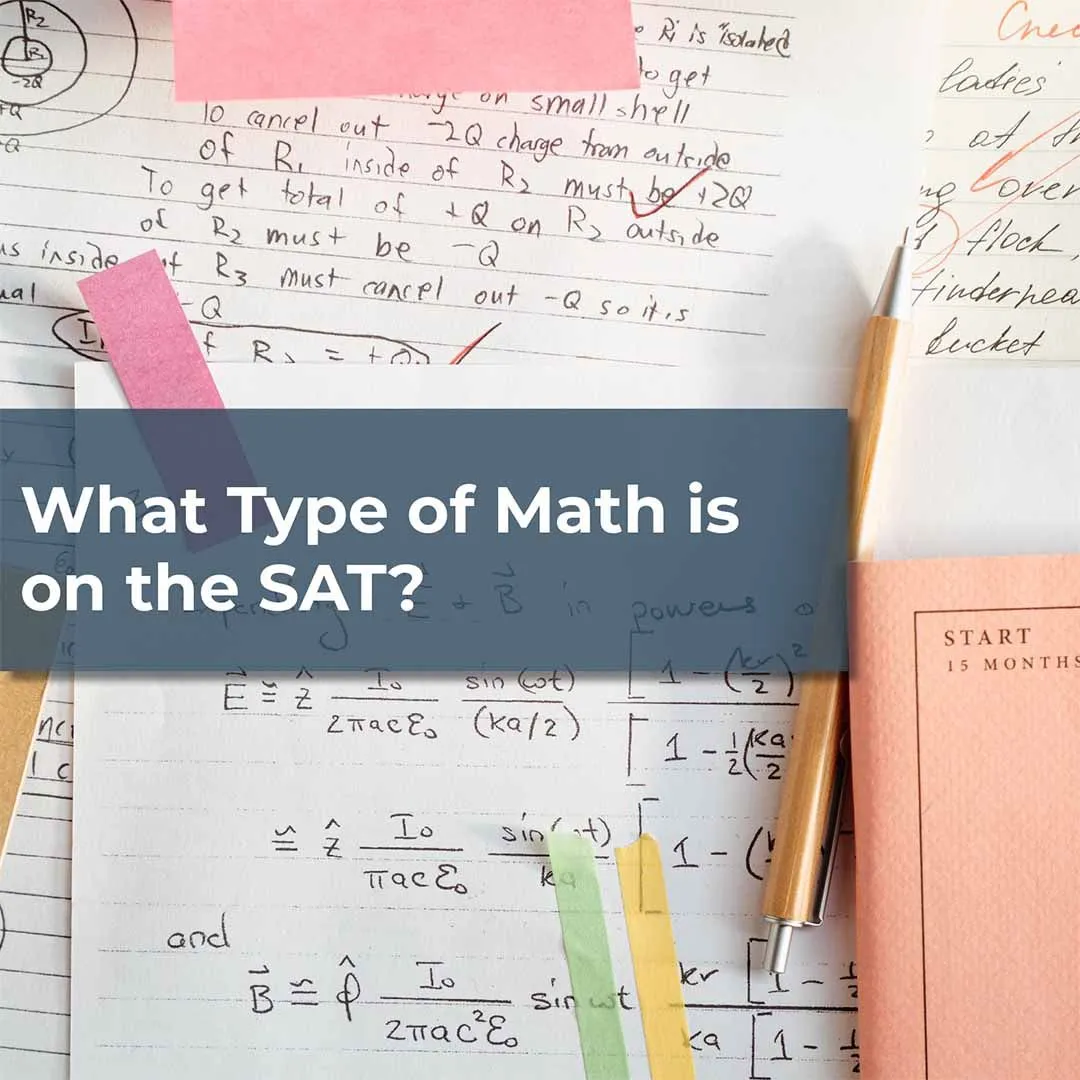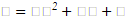Do you know what type of math is on the SAT? To Know more SAT Math and Digital SAT please visit our website# What Type Of Math Is On The SAT ?

If you’re just now starting or thinking about preparing for the SAT, chances are you’re wondering which types of questions are asked on the SAT for math and reading. This article will cover math, but if you want an in depth look at reading strategies and tips to answer the questions, click here!

Half of your total points are going to be from the math section so it's important to be prepared for what you’ll be tested on.

Knowing what kind of math is on the SAT will allow you to focus and streamline your prep so that you don’t waste time preparing for concepts that won’t show up on the exam.

### Here’s a breakdown of what the SAT math section looks like:

The math concepts on the SAT are designed to test your progression in high school level mathematics. The math portion is split into two subsections, one with no calculator and another with calculator allowed.

Now, you might be asking yourself, “how many math questions are on the SAT?” The no calculator portion is 20 questions long, and you are given 25 minutes to complete all questions. The calculator portion is 38 questions long and you are given 55 minutes to complete it. Although it is called the calculator section, you can solve all of the questions without a calculator if you need.

The total raw score you can get for the entire SAT math portion is 58. This raw score is then scaled to a score out of 800, which is determined by the College Board’s scoring system which is known as equating. To learn more about equating, click here to read about some myths about standardized testing! But if you want to know what specifically the SAT math categories are, keep reading below!

### Categories of the SAT math section:

The SAT math section is broken down through four major concepts that make up all of the questions you will see on the exam. These concepts are tested in both the calculator and no calculator section of the SAT.

Here are the four categories in which you will be tested on: Heart of Algebra, Passport to Advanced Mathematics, Problem Solving & Data Analysis, and Additional Topics in Mathematics.

Those are very general and unclear names, so let's break down what they actually mean.

 SAT Concept What it means Percentage of Questions Heart of Algebra Linear Equations and Inequalities, systems of linear functions, linear word problems, linear graphing, absolute value 33% Passport to Advanced Mathematics exponents and radicals, exponential functions, polynomials and nonlinear functions, rational expressions and equations, quadratics, linear and quadratic systems. 28% Problem Solving & Data Analysis units, ratios, proportions, rates, percentages, data distribution, probability, graphs and tables, experimental design 29% Additional Topics in Mathematics Geometry (angles, triangles, right triangles, circles, volume), Trigonometry, Radians, Complex and Imaginary Numbers 10%

Now, let's take a deeper look at what these actually mean.

### Heart of Algebra

This is the bulk of the math SAT and it's a section that most students are very familiar with. Heart of Algebra are questions that challenge students with lines and inequalities. This can include functions and lines, think y=mx+b, as well as graphing lines and figuring out where two lines meet.

Here are some concepts to review within the Heart of Algebra:

• Match an image of a graph to a linear equation, and vice versa.
• Finding and Determining Slope from tables and equations
• Finding the midpoint of a line using the midpoint formula.
• Using lines to represent real life scenarios or word problems.
• Solving for Variables in a linear equation.
• Parallel and perpendicular lines.
• Real-world situations with and inequalities.
• Graphing inequalities.
• Solving systems of linear equations and inequalities.
• Graphing systems of linear equations and inequalities.
• Graphing and solving equations with absolute values.

This, for most students, is the hardest part of the SAT math section. This tests your ability to work with and model non linear equations and relationships. This includes quadratics, rational functions, and polynomials.

Most of what you’ll encounter in this section is based on quadratic equations. As a quick refresher, these are represented as y=ax2+bx+c.

Here are some question types that you might see under this category:

• Matching graphs of parabolas to quadratic equations and vice versa.
• Factoring quadratic equations. While the SAT won’t ask you to use a specific method, here are some strategies you can use to factor
• Factoring normally.
• Factoring differences of perfect squares.
• Factoring with the Quadratic Formula.
• Factoring by grouping.
• Factoring by completing the square
• Finding vertices of nonlinear equation
• Solving systems of equations with at least one quadratic equation
• Polynomial division
• Solving with the discriminant
• Modeling exponential growth/decay from a word problem.
• Graphing exponential equations.
• Solving exponential equations.
• Exponent rules.
• Solving radical equations (and avoiding extraneous solutions).
• Rationalizing a denominator with roots or imaginary numbers
• Common denominators for rational expressions.
• FOIL
• Simplifying.

### Problem Solving & Data Analysis

This section tests your ability to solve statistical analysis as well as create complex relations between data. They aren’t necessarily linear or nonlinear, but instead tests your data analysis skills. Here are some of the problem types you might encounter on the SAT.

1. Performing Unit Conversions
2. Solving Equations with ratios and proportions
3. Percentage calculations
4. Finding original value from percentage change and final value
5. Finding final value from original value and percentage change

Understanding basic measures of center:

1. Mode, Range, Medium
2. Finding Mean / Average
3. Solving Mixtures
4. Solving equations with mean/average value
5. Standard Deviation
6. Probability
7. Probability of a particular outcome
8. Graphs and tables
9. Line of best fit
10. Scatter plots, bar graphs, histogram, stem and leaf plots, and box and whisker plots.
11. Understanding experimental design
12. Sampling BIas
13. Study credibility metrics

This is pretty broad but these concepts cover everything that is not above but can be tested on the SAT. They are made up of different areas of mathematics from geometry to complex numbers. Here are some of the possible questions:

• Triangles
• Using Pythagorean theorem to find right angles
• Trigonometry of right triangles
• Solving similar triangles
• Solving 30-60-90 and 45-45-90 triangles
• Finding area of triangles
• Triangle angle sum theorem
• Matching sides to angles
• Triangle Inequality Theorem
• Circles
• Radius and Center of Circle using equation of circle
• Finding area and circumference
• Converting between radians and degrees
• Finding Area of a Partial Circle
• Finding Angle measurements
• Miscellaneous Geometry
• Area and perimeter of rectangles and squares
• Finding the area of other polygons
• Finding missing angles in transversals
• Interior Angle Measurements
• Volume of regular shapes
• Comparing area and volume of different shapes

Additionally, while it only usually appears once per the exam, if you’re aiming for that perfect score, you should know how to use imaginary numbers and i.

### How to study these topics

Now that you know everything about all math topics on SAT, how should you actually go about studying for the exam? There are many different strategies out there for how you can study, but make sure to use Park Tutoring’s strategies to get the best results.

One popular way to study is to do an SAT Math Problem of the Day. This allows you to study up on your concepts with a simple problem, even when you’re busy. Even doing just one problem per day is enough to keep your skills sharp for the exam, and make sure that you’ll be able to keep these concepts fresh in your mind.